# TRANSFER FUNCTIONS OF CONTROL SYSTEM

This article is about TRANSFER FUNCTIONS OF CONTROL SYSTEM and focusing to the engineers, technicians and supervisors. You will find lot of documents related to this article. Just navigate our website www.paktechpoint.com  and find more articles. Please! Do not forget to subscribe our You tube channel also. Thanks in Advance. PLEASE SUBSCRIBE OUR PAKTECHPOINT YOUTUBE CHANNEL

## TRANSFER FUNCTIONS OF CONTROL SYSTEM

#### CONTENTS TO PROCEED

• System
• Classification of systems
• System analysis
• Mathematical model
• Linear time invarient system
• Transfer function (definition, properties, example)
• Block diagrams ( elements, advantages, example)
• Rules for block diagram reduction + example
Transfer function + block diagram of mechanical system

### SYSTEM

“A process that results in transforming input signals into output signals.”
In studying the behavior of a system, the procedure is to model mathematically each element of the system and then to consider the interconnection of elements.
The result is described mathematically either in the time domain or in the frequency domain.

### CLASSIFICATION OF SYSTEMS

• continuoustime or discretetime.
• linear or nonlinear.
• time invariant or time varying.
• causal or noncausal.
• stable or unstable.
• deterministic or nondeterministic.

### Discrete systems

If a signal is known only at certain discrete instants of time, then it is known as a discrete time signal.

### Linear and Non-Linear Systems

An important criterion that distinguished nonlinear systems from linear systems is the principle of superposition. Most natural systems are nonlinear.

### Causal and Non-Causal Systems

A causal or non anticipatory system is one in which the output xo(t1) at any arbitrary instant t1 depends on its input xi(t) in the past up to and including t = t1.

### Stable and Unstable Systems

A system whose response either oscillates within certain finite bounds or grows without bounds is regarded as unstable. If for every bounded input the output is bounded, the system is said to be stable.

### Deterministic and Stochastic Systems

• In the deterministic case, the signals and the mathematical model of a system are known with certainty and the time behavior can be reproduced by repeated experimentation.
• In the stochastic case this not possible due to uncertainty that exists either in its model parameters or in its signals or in both. The values of the signals or the variables occurring in the system can only be estimated. The results are presented as expected values together with the bounds of error.

## CONTROL SYSTEM ANALYSIS AND DESIGN

There are two general approaches controls analysis and design.

MATHEMATICAL APPROACH

• Representation in equations
• Results in equations
• Consider all possible inputs

#### SIMULATION OR EXPERIMENTAL APPROACH

• Precise
• Results limited to system studied
• Consider one speciﬁc input

### MATHEMATICAL MODEL

A mathematical model of a system is defined as “A set of equations that represent the dynamics of the system accurately, or  at least fairly well” A mathematical model is not unique to a given system. A system may be represented in many different ways and therefore may have many mathematical model.

### LINEAR TIME INVARIENT SYSTEM

The defining properties of any linear time invariant system are, of course, linearity and time invariance.
• Linearity means that the relationship between the input and the output of the system satisfies the superposition property.
• Time invariance means that whether we apply an input to the system now or T seconds from now, the output will be identical.

### PRINCIPLE OF SUPER POSITION

This principle states that the response produced by the simultaneous application of two different forcing functions is the sum of two individual responses. Hence, for the linear system, the response to several inputs can be calculated by treating one input at a time and adding the results.

### LINEAR TIMEINVARIANT SYSTEM DESCRIPTION

A Linear TimeInvariant system LTI can be described in two ways:
A Linear Constant Coefficient Differential Equation (LCCDE)
A Transfer Function: Every system described by an LCCDE has a TF (and vice versa)

## THE TRANSFER FUNCTION

“The Transfer Function is the ratio of the Laplace Transform of the output signal over the Laplace Transform of the input signal.  The transfer function is commonly used in the analysis of singleinput singleoutput analog electronic circuits.

The term is often used exclusively to refer to linear, time invariant systems (LTI). Most real systems have non linear input/output characteristics, but many systems, when operated within nominal parameters have behavior that is close enough to LTI system theory an acceptable representation of the input/output behavior.

#### MATHEMATICAL REPRESENTATION

or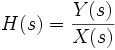For continuous time input signal and output , the transfer function is the linear mapping of the Laplace transform of the input X(s), to the output Y(s).
Where H(s) is the transfer function of the LTI system.

### PROPERTIES OF TRANSFER FUNCTION

Components of a Transfer Function:
All information about the signals is included in the Laplace Transforms U(s) and Y(s).
All information about the system is included in the transfer function H(s).
When we evaluate the transfer function we are evaluating the response of the system to any specific input or out put.
We can use H(s) and design a controller without ever knowing U(s) or Y(s).
The transfer function has units of gain.
A transfer function is a rational polynomial.
Any algebra we can do with a function of s, we can do with a Transfer function.
Transfer functions depend on linearity; non linear systems do not have a transfer function.
The transfer function does not provide any information concerning the physical structure of the system. The transfer function of many physically different systems can be same.
If the transfer function of a system is known, the output or response can be studied for various forms of inputs with a view towards understanding the nature of the system.
EXAMPLE
The transfer function is obtained by considering the torque as the input and angular displacement as the output. To derive the function we proceed with the following steps:
1. Write the differential equation for the system.
2. Take the laplace transform of the differential equation, assuming all initial conditions are zero.
3. Take the ratio of output to input. This ratio is the transfer function.Applying Newton’s second law to the system we get
J d² Θ /dt²= T
Taking laplace transform on both sides,
J s² Θ(s) = T(s)
The transfer function of the system is thus obtained as
Transfer function = Θ(s)/T(s)  = 1 / Js²

### Block Diagrams:

“A block diagram of a system is a pictorial representation of the functions performed by each component and of the flow signals. Such a diagram depicts the interrelationship between components.”

### ELEMNTS OF BLOCK DIAGRAM

#### BLOCK

• All system variables are linked to each other through functional blocks.
• The block is a symbol for the mathematical operation on the input signal to the block that produces output.
• The transfer function of the components are usually entered in the corresponding blocks.

#### SIGNAL

• The blocks are connected by arrows to indicate the direction of low of signals.
• The signals can pass only in the direction of arrows.
• The arrowhead pointing towards the block indicates the input , and the arrow head leading away from the block represents the output . Such arrows are referred to as signals.

#### SUMMING POINT OR JUNCTION:

• The summing point is represented by a circle. The signal out of the summing junction is equal to the sum of signals into the junction, with the sign of each component determined by the sign placed near the arrow head of the

component.

#### BRANCH POINT:

• A point from which signal from a block goes to other blocks or summing points is called branch point.

### ADVANTAGE OF BLOCK DIAGRAM

1. It is easy to form the block diagram for the entire system by merely connecting the blocks of components according to signal flow.
2. It is possible to evaluate the contribution of each component to the overall performance of the system.
3. In general the functional operation of the system can be visualized more readily by examining the block diagram than by examining the physical system itself.

### PROCEDURE FOR DRAWING A BLOCK DIAGRAM:

Following procedure is adopted:
• Write the equations that represent the dynamic behavior of each component.
• Take the Laplace transform of theses equations, assuming zero initial conitions.
• Represent each Laplace transformed equation individually in a block form.
• Finally assemble the elements into a complete bock diagram.

### RULES FOR BLOCK DIAGRAM REDUCTION:

1. Moving summing point to right of block: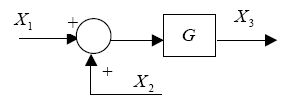When the summing point is moved towards the right of the block, the feedback function is multiplied with the block or the transfer function.
2. Moving summing point to left of block: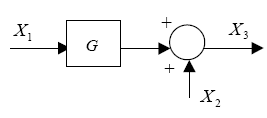When the summing point is moved towards the left of block, the feedback function is divided by the block or transfer function.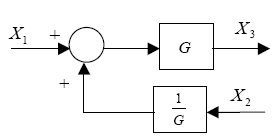3. Moving branch point to right of block: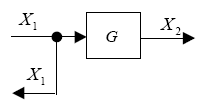When the branch point is moved towards the right of block, the output is divided by the block or transfer function.4. Moving a branch point to the left of block: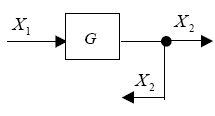When the branch point is moved towards the left of block, the output is multiplied by the block or transfer function.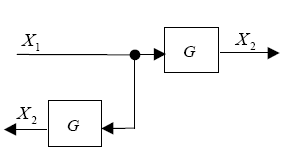5. Multiplication of series transfer functions: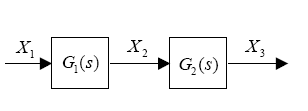from definition of transfer function
X3 = G2(s) X2 …………….1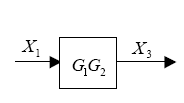X2 = G1(s) X1 …………….2
Put 2 in 1
X3 = G2(s) G1(S) X1
6. Addition of parallel transfer functions:
The left block diagram gives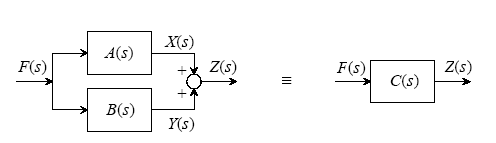Z(s) = X(s) + Y(s)
= A(s) F(s) + B(s) F(s)
= [ A(s) + B(s) F(s)…………….1
and the right block gives
Z(s) = C(s) F(s)…………………….2
Comparing 1 & 2 we get,
C(s) = [ A(s) + B(s) ]
7. Eliminating feedback loop: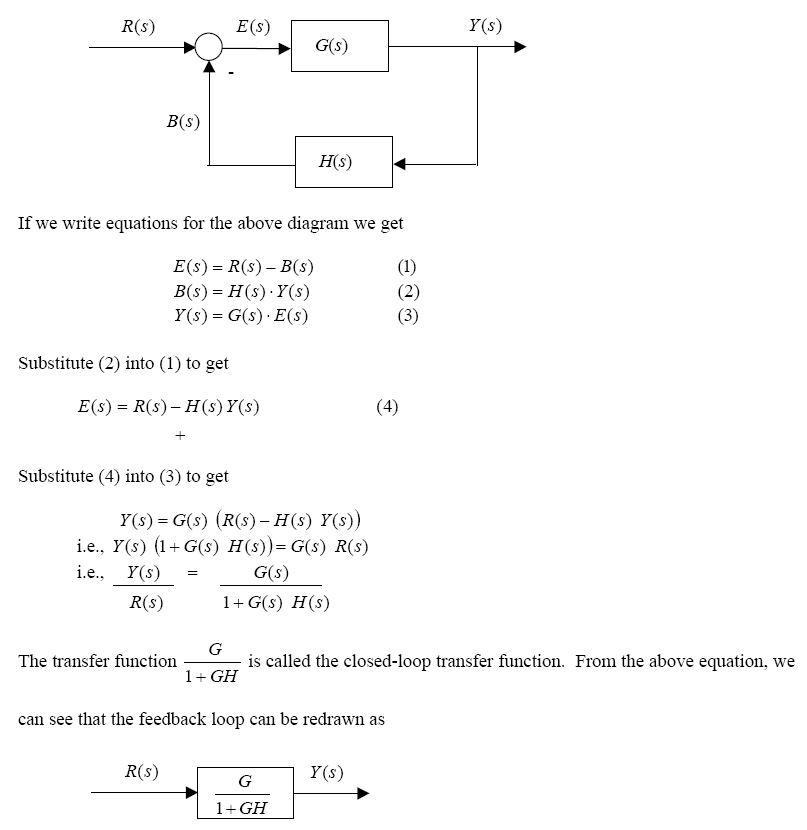EXAMPLE OF BLOCK DIAGRAM REDUCTION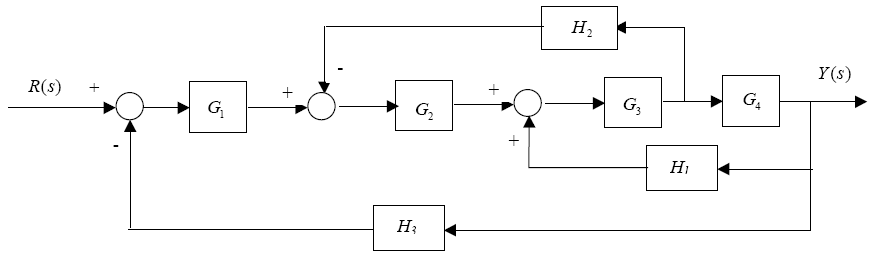First, let’s move H2 behind block G4 so that we can isolate the G3 – G4 – H1 feedback loop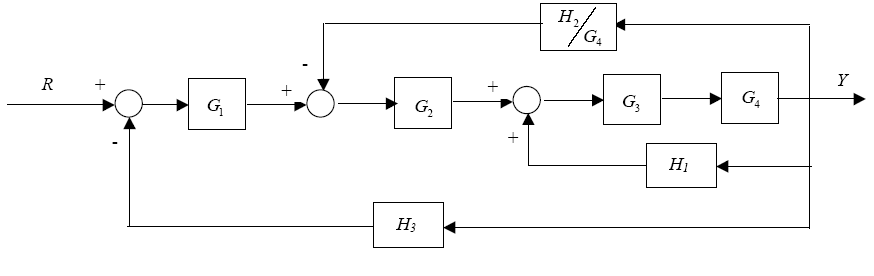Now, we can eliminate the G3  G4  H1 loop.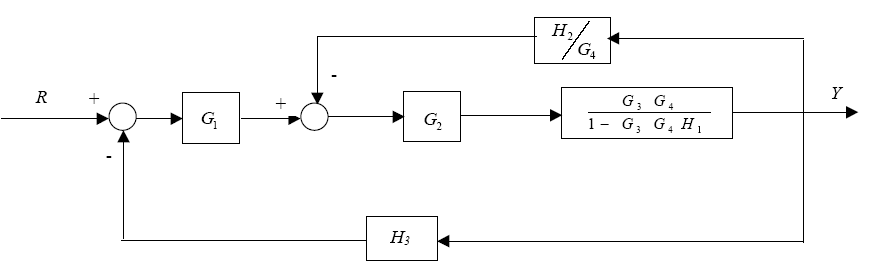Now, eliminate the inner loop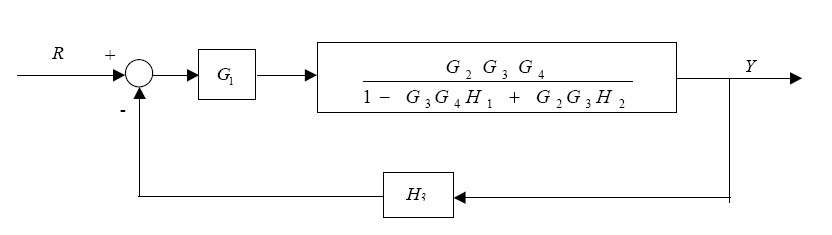Therefore, the equivalent diagram is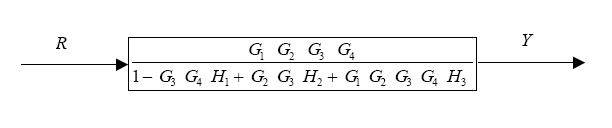### EXAMPLES OF DERIVING TRANSFER FUNCTION AND BLOCK DIAGRAMS

Consider a simplified model of an automobile suspension system of one wheel, with M1 the mass of the wheel, and K2 the elasticity of the tire. Note that two equations must be written, since two independent displacements exist.
First forces are summed about mass M1
M1 dx1/dt² =- B (dx1/dt – dx2/dt ) – K1( x1 -x2)
Next the forces on M2 are summed. Three elements are connected to M2 in addition to the applied force. Hence
M2 d²x2/dt²= f(t) – B( dx2/dt – dx1/dt) – K1 (X2  X1) – k2x2
Now taking the laplace transform of these equations yields
M1 s² X1(s) + B [s X1(s) – s X2(s) ] + K1 [ X1(s) – X2(s)] = 0
M2 s² X2(s) + B [ s X2(s) – s X1(s)] + K1 [X2(s) – X1(s) ] + K2X2(s) = F(s)
Simplifying the first equation,
M1 s²X1(s) + BsX1(s) – BsX2(s) + K1 X1(s) – K1X2(s) = 0
[ M1 s² + Bs + K1] X1(s) = [ Bs + K1] X2(s)
X1(s) =[ Bs + K1] X2(s) [ M1 s² + Bs + K1]
X1(s) = G1(s) X2(s)……………………………1
Where G1(s) is the transfer function replaced for convenience.
Now solving the other equation for X2(s) we get,
M2 s² X2(s) + Bs X2(s) – Bs X1(s) + K1 X2(s)  K1X1(s) + K2X2(s) = F(s)
[ M2 s² + Bs + K1 + K2] X2(s) = [ Bs + K1]X1(s) + F(s)
X2(s) = [ Bs + K1] X1(s) +F(s) [ M2 s² + Bs + K1 + K2]
X2(s) = [ Bs + K1] X1(s) + F(s)
[ M2 s² + Bs + K1 + K2] [ M2 s² + Bs + K1 + K2]
X2(s) = G2(s) F(s) + G3(s) X1(s)………………….2
On the basis of equations 1 & 2 we can draw the block diagrams, by identifying the inputs, outputs, and the transfer function. As shown below :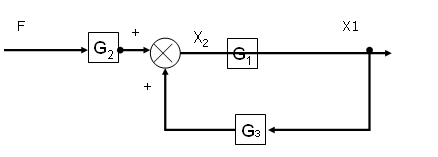By eliminating the feedback loop using rule mentioned above, the block diagram reduces to following
T(s) =  X1(s) =  G1(s) G2(s) . F(s) 1- G1(s) G3(s)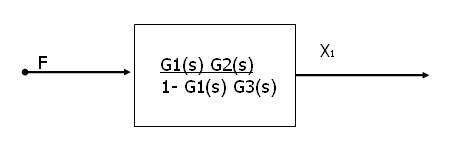## 8 thoughts on “TRANSFER FUNCTIONS OF CONTROL SYSTEM”

1.Thanks for every other informative blog. The place else could I get that kind of info written in such a perfect manner? I’ve a undertaking that I’m simply now working on, and I’ve been on the glance out for such information.

2.My family every time say that I am wasting my time here at net,but I know I am getting experience daily by reading such pleasant articles.

3.Love Diagrams and all about them !